# Performance anxiety

In our last post, we took a quick look at building a portfolio based on the historical averages method for setting return expectations. Beginning in 1987, we used the first five years of monthly return data to simulate a thousand possible portfolio weights, found the average weights that met our risk-return criteria, and then tested that weighting scheme on two five-year cycles in the future. At the end of the post, we outlined the next steps for analysis among which performance attribution and different rebalancing schemes were but a few.

For this post, we’ll begin analyzing performance. Before we start we want to highlight a few points. First, over the last few posts we’ve included python versions of the analysis and graphs we produce in R. While we’ll stop flagging the presence of the python code going forward, we will continue to present it after the R code at the end of the text. If you find seeing the python code useful or have questions on reproducing our results, let us know at the email address below. We respond!

Second, we want to point out that the simulation method we used for the last post and this one is biased toward an average allocation. In other posts, we used a different, “hacky” method to allow for more extreme allocations. One kind reader suggested a more elegant solution based on the Dirichlet distribution. These different methods deserve a post on their own to explain what they’re doing and why you might prefer one over the other. That will have to wait. Nonetheless, we wanted to mention it in case anyone looked closely at the code. We’re opting for simple methods to drive our illustrations before moving into more complicated expositions. Cave paintings before Jackson Pollock if you will.

Third, if you’ve read some of the past posts and are scratching your head, wondering why the heck we haven’t graphed the efficient frontier or shown the tangency portfolio yet, don’t worry. We’ll get there! We want to build some intuitions for non-finance folks first. We also have some reasons for avoiding these tools that we’ll explain when we finally tackle them. On the other hand, if the efficient frontier sounds like a destination for Captain Kirk’s evil twin, we’ll explain it all soon enough. Now let’s boldly move on to the post.

When we looked at the two test periods for our portfolio, we noticed we significantly beat our return constraints in the first period, yet missed them in the second. Given these results, it might be a good idea to try to understand what were the sources of performance in the first period and what that might suggest about our allocation decisions for the next.

#### Performance by asset

Let’s bring in the data, graph our portfolio simulations, and then look at the first performance period. Recall, our portfolio is comprised of four indices that encapsulate the major asset classes: stocks, bonds, commodities (gold in this case), and real estate. We pulled this data from the FRED database. Here’s the simulation of 1,000 potential portfolios shaded for the Sharpe ratio, based on the average risk and return of each asset for the five-years ending in 1991. See the first post for details on our choice of data and length of time series.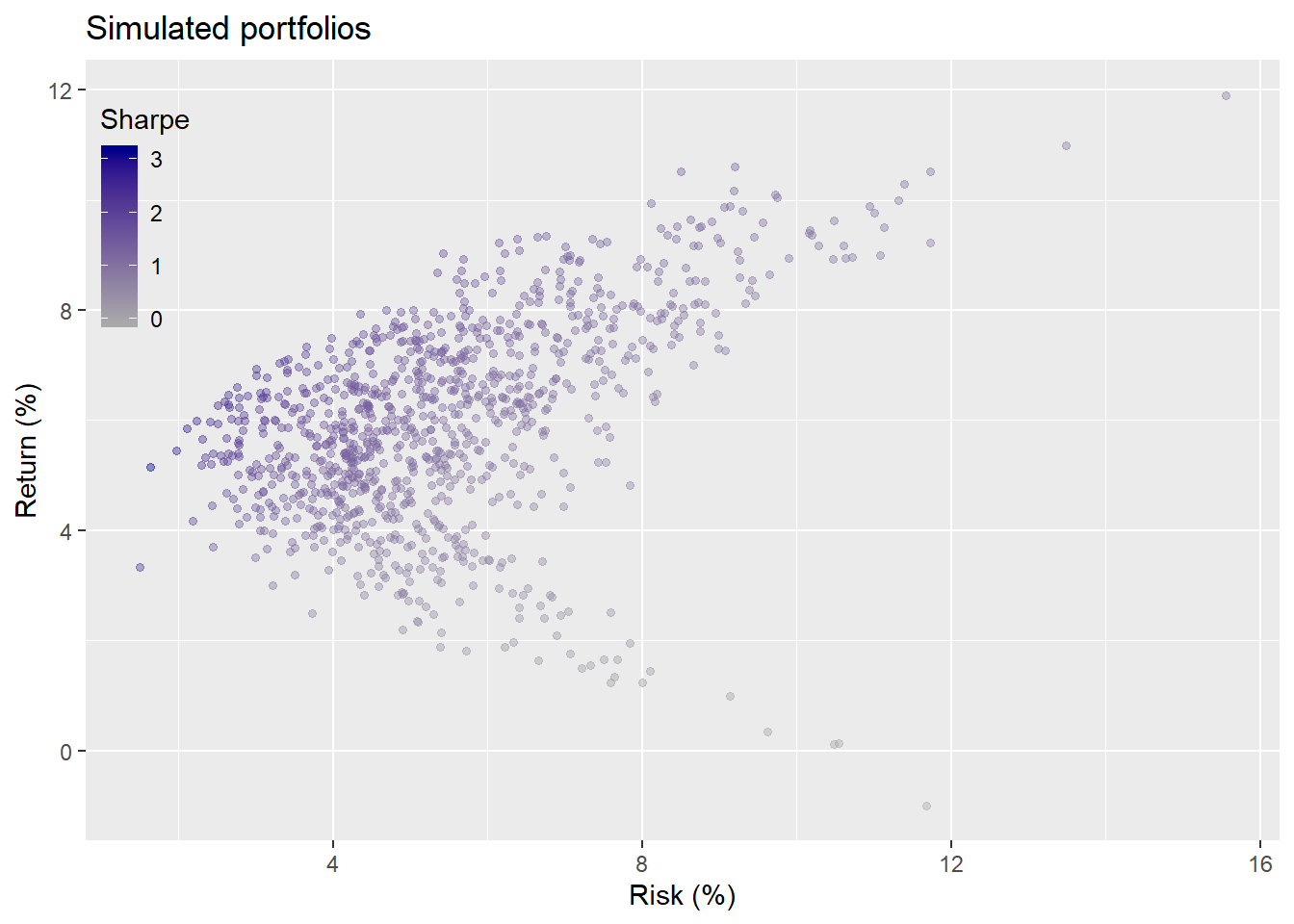Here’s the proposed weighting based on a required return of not less than 7% and a risk of not more than 10%This how that portfolio performed relative to a 1,000 simulated portfolios in the first five-year test period: 1992-1997.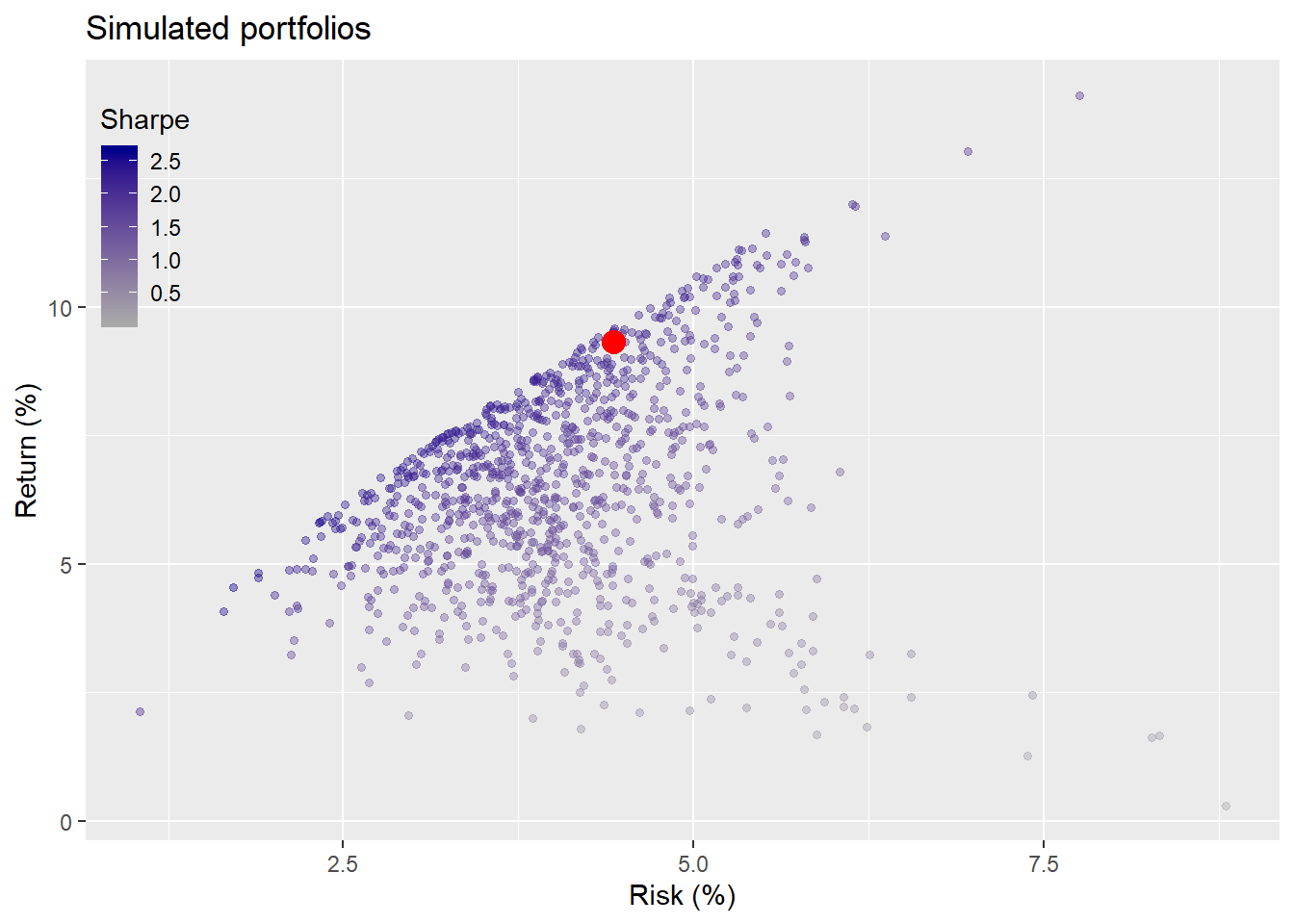What did each asset class contribute to the overall portfolio performance in the first test period?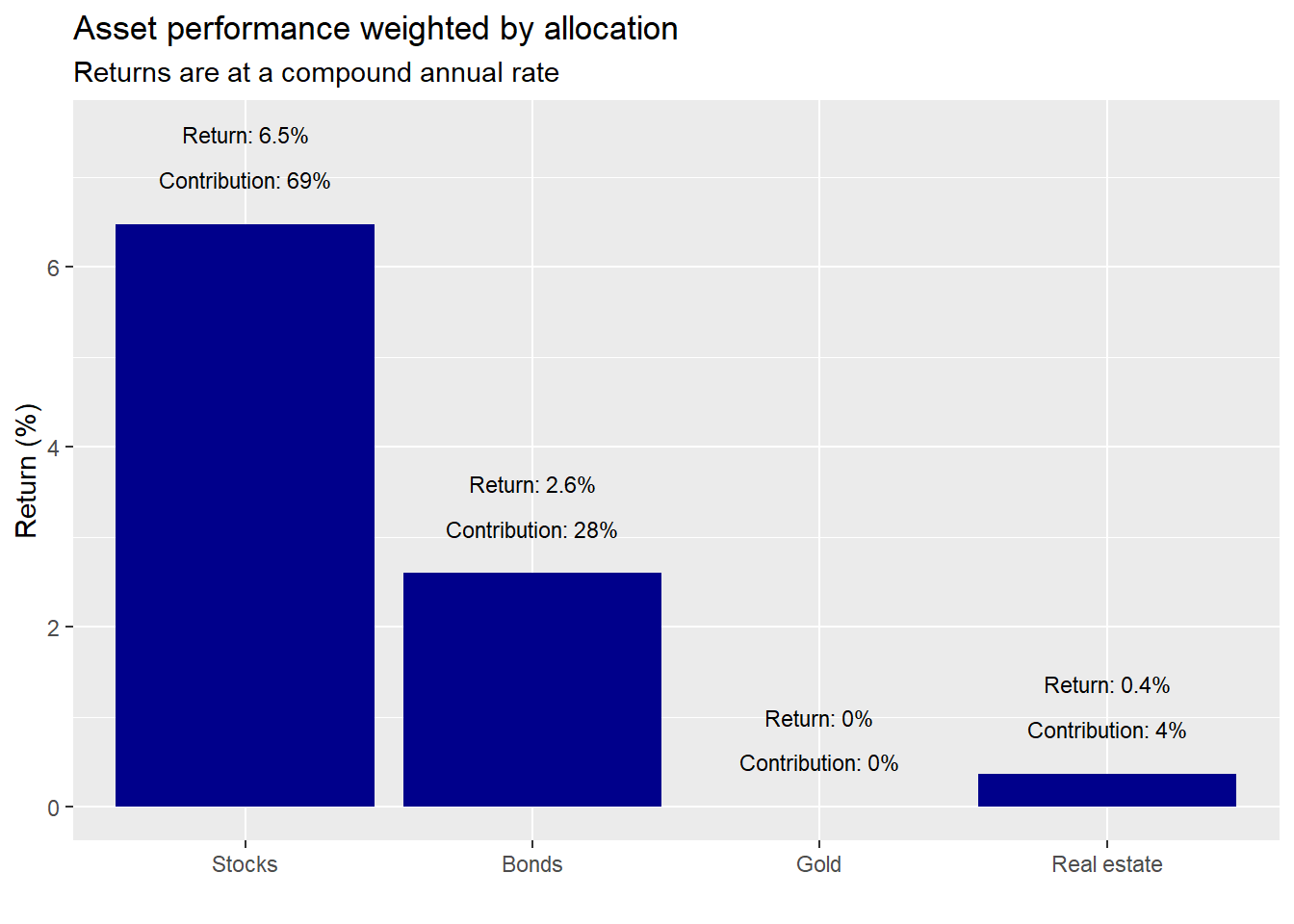We see that stocks were the biggest contributor while gold did nothing. Let’s look at risk contribution.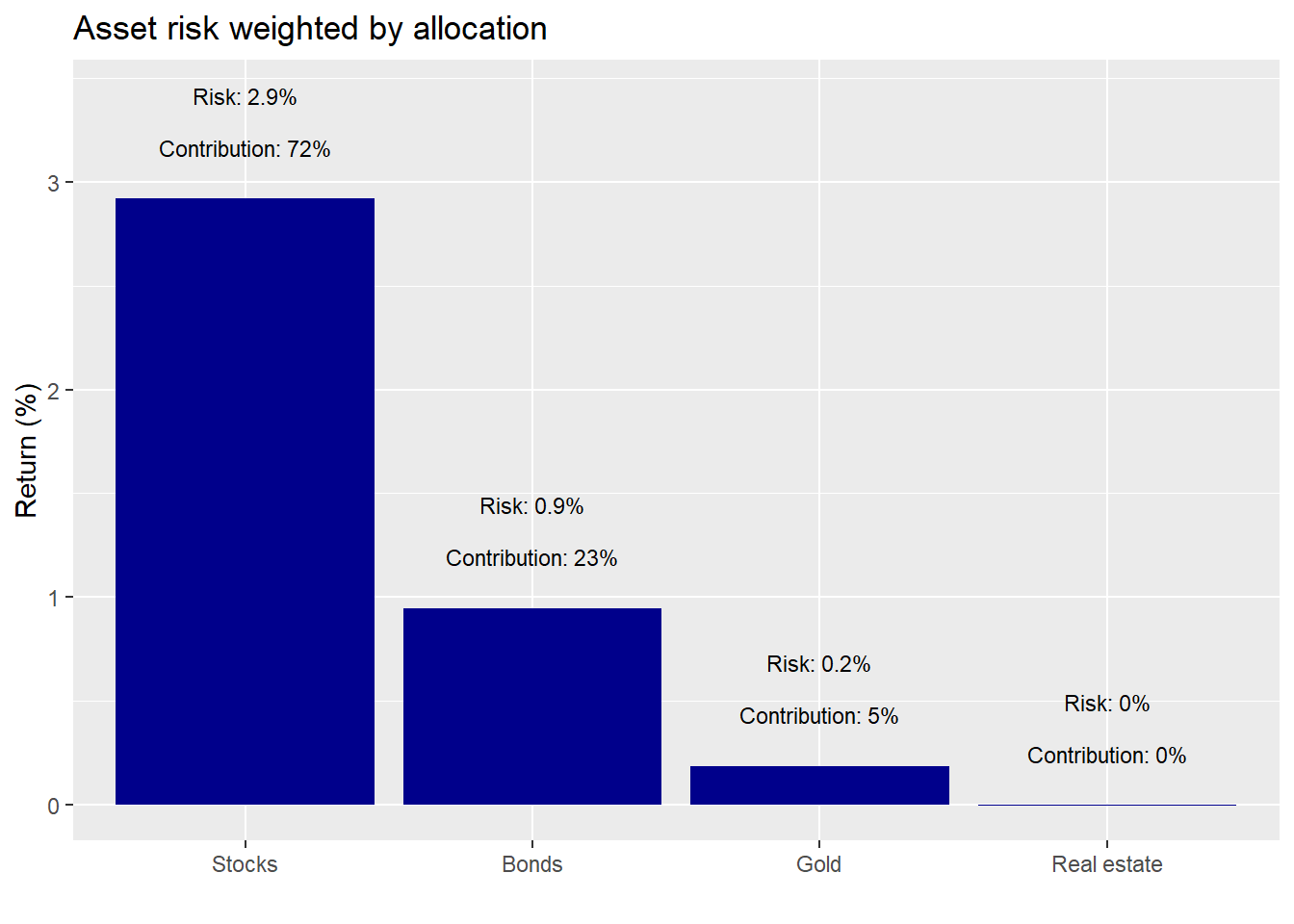Here again stocks sported the biggest contribution. Real estate’s “zero” contribution is an artifact of rounding. Still it enjoyed very low volatility and low correlation with the other asset classes. Hence, a de minimis effect on portfolio volatility, an important finding.

A couple things should stand out about these results. First, it’s all about stocks and bonds. Both were the biggest contributors to returns and risk. Despite, a combined initial weight of about 67%, these two assets were responsible for about 96% of the return and 95% of the risk.

Second, comparing contributions, we see that stocks contributed slightly more to risk than they did to return. But the differences are modest. Alternatively, bonds contributed relatively more to returns than they did to risk.

Should we be surprised by these results? The original weighting for stocks was 37% vs. bonds at 30%, real estate at 22% and the remainder in gold. The stocks’ high contribution was due to their overall strong performance during the period. We nearly met our return constraints with one asset! However, since we didn’t rebalance, it also means that by the end of the period, stocks made up significantly more of the portfolio than they did at inception as shown in the graph below. Portfolio risk would be meaningfully driven by stocks going forward.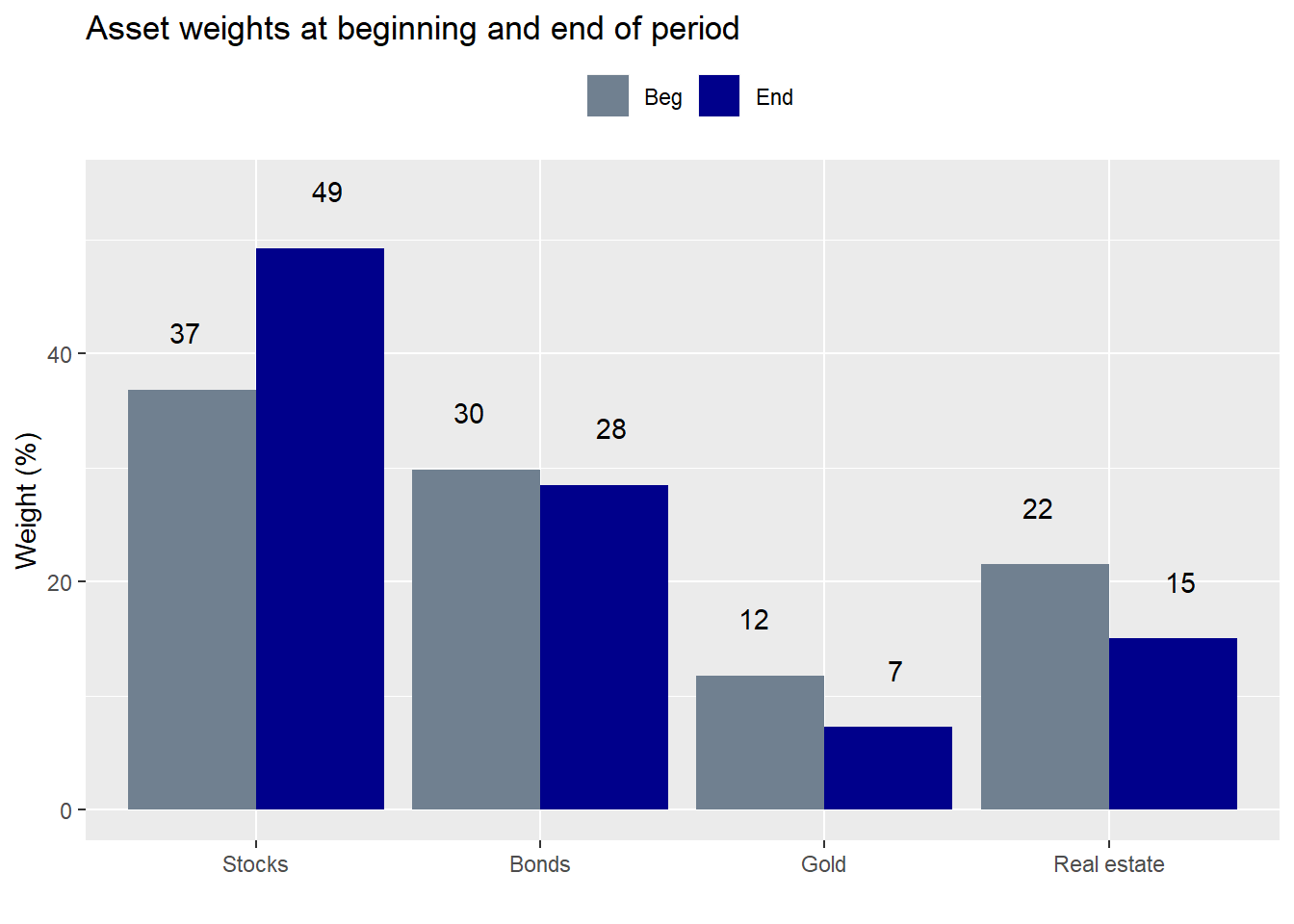Recall that our constraints were not more than 10% risk and not less than 7% return on an annual basis. In the last post we decided not to change the original weighting for the next five year period, although we did compare it to a different weighting scheme. Indeed, when we re-ran the the weighting calculation to achieve our target constraints incorporating the recent performance, results advised a return to original allocation. What we didn’t mention was that returning to the old weighting scheme, implies rebalancing, which would entail transaction costs. If we had left everything as is at the end of the first five-year period (purple dot), here is how the portfolio would look compared to rebalancing (red dot) and a 1,000 simulations on the second five-year period.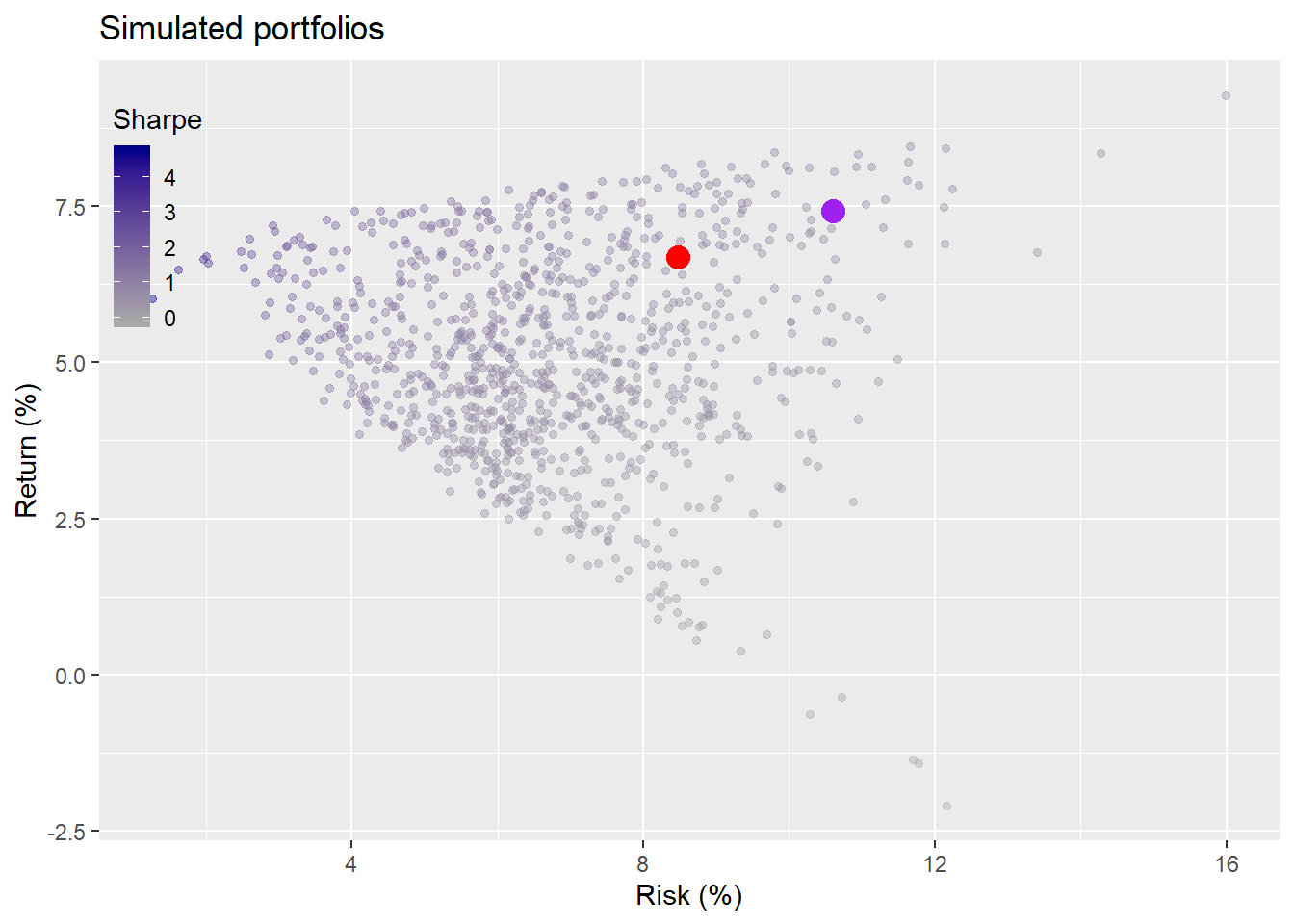The rebalanced portfolio yielded a modestly worse return, but a much better risk profile. Of course, in both cases, those portfolios were dominated by others. That is, there were other allocations that generated a higher return for the same level of risk. The rebalanced portfolio kept risk within the constraints even though returns missed the target. The non-rebalanced portfolio overshot the risk constraint. Still, only 14% of the portfolios met or exceeded the return constraints, while 94% of the simulated portfolios met the risk constraints.

In this case, rebalancing might not have been a bad idea. However, to analyze it properly we’d need to estimate transaction costs, including tax. Coding such effects warrants a series on its own. But back of the envelope suggests the following. The portfolio grew almost 58% so if we redeployed 12% of ending capital (the amount by which stocks are greater than their target weight), that equates to almost 30% of the growth being taxed.1 Assuming long term capital gains tax rates of 20%, that would equate to a 6 percentage point drag on cumulative performance.

So even if we like the lower risk conferred by rebalancing, it needs to be worth the lower potential return due to taxes. How might we analyze that? One way might be to calculate the improvement in the Sharpe ratio. By returning to the original weighting, the Sharpe ratio would improve by 17 percentage points, based on the returns of the prior 10-year period. Seems like a lot, but even if the return per unit of risk improves are we taking enough risk to meet our return targets.2 Sharpe ratios don’t feed the kids as they say. Of course, if the portfolio is operating within a tax-free environment, that point is moot. Whatever the case, let’s think about what the performance results suggest.

First, we’d want to consider the financial assets vs. the real ones. The financial assets (stocks and bonds) are generating the bulk of the return and risk. Gold did little, though it didn’t add risk to the portfolio. Real estate was more interesting as it did contribute modestly to the return, but contributed very little to risk, mainly due to its low to negative correlation with financial assets. Should we dispense with gold altogether? Its negative correlation with financial assets yields some salutary benefits in terms of risk. But returns leave a lot to be desired.

Second, do we want to maintain the same weights on the financial assets? If we had a taxable, static portfolio we’d need to consider whether the tax impact was worth the lower risk, as discussed above. However, if we received regular inflows of capital, then we could deploy it to return to the target weights without paying taxes. Of course, that would expose us to some additional risk; that is, how long we’d be willing to sustain off target allocations and how much timing risk we’d want to endure. Even if we wanted to bring our allocations back to their original weights, there might be a timing mismatch between when we had new funds to allocate and whether the price we might pay still aligned with our original risk-return projections.

Alternatively, rather than selling winners to buy losers, what if we sold the losers and redeployed the capital into more attractive assets, entirely eliminating exposure to one asset class?3 Recall how we flagged how simple our simulation was? Well, it was even more simplistic than that, since we didn’t include an option to invest in only a subset of the assets. Excluding assets adds another wrinkle to the analysis and to the growing list of posts we need to write. But let’s look briefly at how the portfolio would have fared relative to others that excluded some assets.

In the graph below, we simulate 3,000 portfolios for different combinations of assets. That is, a thousand each for four, three, and two asset portfolios.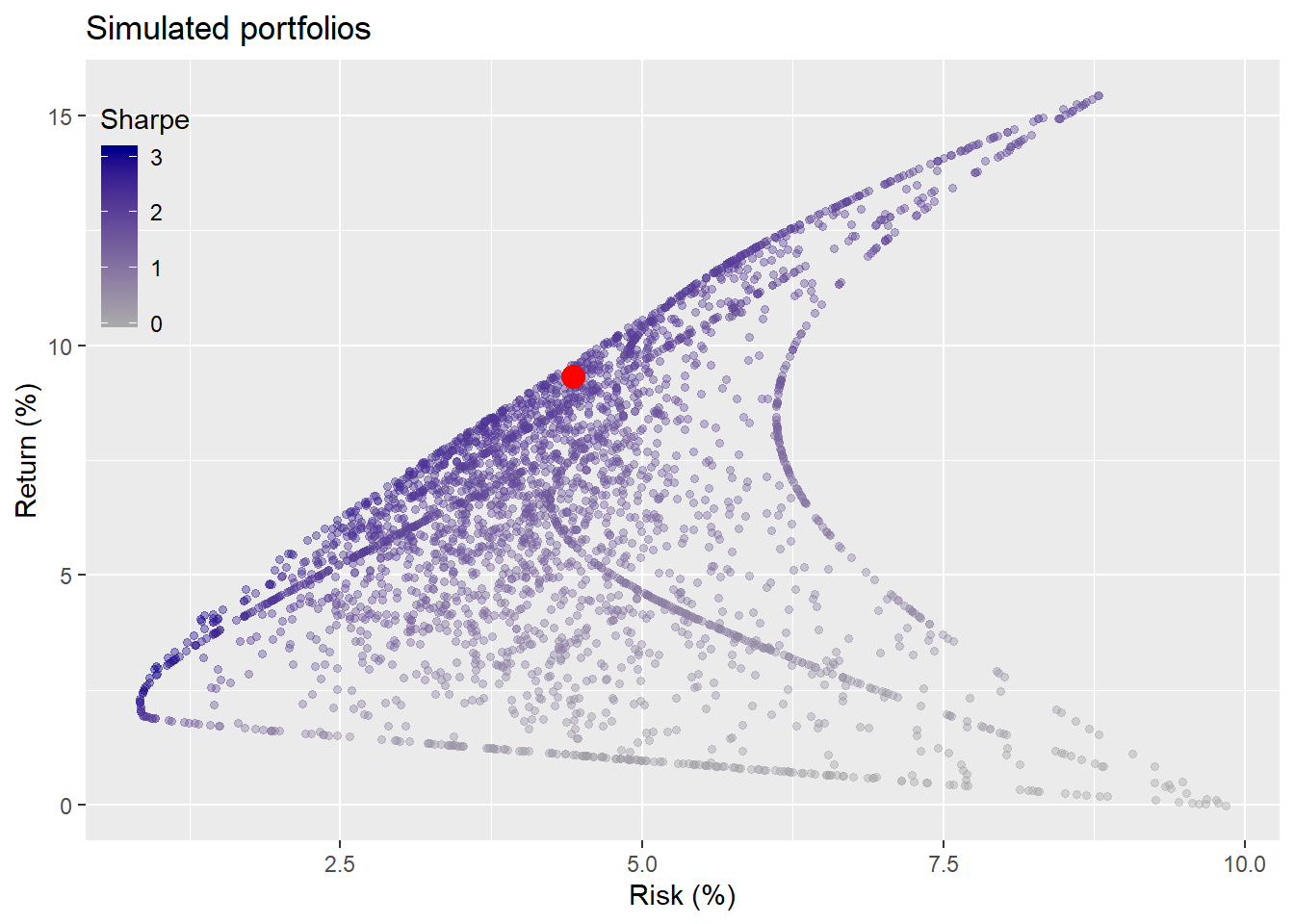As one can see, when we add in the chance to exclude assets, the range of potential outcomes increases significantly. There’s only one way to choose four out of four assets. But there are eleven ways to choose four, three, and two out of four assets.4 The original portfolio lies along the dark band of better risk-return (e.g, higher Sharpe ratio) portfolios. In fact, it’s Sharpe ratio is better than 84% of the portfolios. But about 85% of the portfolios beat our risk-return constraints, so one could have been playing with blunt darts and still have stuck the board.

Where does this leave us? The key is that past performance provides powerful insights, but is only one sample. The range of possible results is vast, and grows multiplicatively when you add even a few reasonable choices.

We see that when only a portion of our assets are responsible for the bulk of meeting our constraints, it’s worthwhile considering removing, or, at least de-emphasizing, the non-contributing assets. Before we do that, we should analyze whether we’re removing them because they performed poorly, yielded results outside the range of likely expectations, or because we’re trying to predict the future. On the first case, poor performance alone doesn’t warrant surgery, as all assets suffer periods of weak returns. If we’re considering exclusion due to results outside of likely expectations, we need to make sure our expectations were well calibrated. Then we need to decide whether to revise our expectations and understand the level of mean reversion present in that asset. Five hundred year floods can happen more than once in a decade. That doesn’t mean the probability that there will be more than one in the next decade has changed.

Finally, if we’re trying to predict the future, do we have a framework or process that gives us a reasonable probability of success at such a fraught endeavor? Even if we’re not, are we implicitly doing so due to behavioral biases because we’re all too human? If we’re only trying to earn a return commensurate with risk, then we have to be careful that we’re not substituting a “belief” about the future for a disciplined process to justify changes to our portfolio

All of these questions refer back to what our expectations were. We looked at historical averages and chose a portfolio based on the average weights that would produce a reasonable risk-return profile. That implicitly assumes the future will look like the past. But as we saw, we outperformed and then underperformed that assumption. Was it reasonable to expect our portfolio to meet those constraints? Just because it did or didn’t shouldn’t we be analyzing performance based not only on what happened but also on reasonable expectations of what could have happened? Sure we assumed implicitly that the future would look like the past, but did it and what might it have looked like otherwise?

Ultimately, we want to know whether our logic was sound regardless of the outcome. We want to make sure we’re wrong for the right reasons, not right for the wrong ones. We’ll look at ways to simulate expectations and analyze our logic in our next post. Until then the R and then python code are below. Enjoy.

#### Load packages ####
suppressPackageStartupMessages({
library(tidyquant)
library(tidyverse)
})

sym_names <- c("stock", "bond", "gold", "realt", "rfr")

port_sim <- function(df, sims, cols){

if(ncol(df) != cols){
print("Columns don't match")
break
}

# Create weight matrix
wts <- matrix(nrow = sims, ncol = cols)

for(i in 1:sims){
a <- runif(cols,0,1)
b <- a/sum(a)
wts[i,] <- b
}

# Find returns
mean_ret <- colMeans(df)

# Calculate covariance matrix
cov_mat <- cov(df)

# Calculate random portfolios
port <- matrix(nrow = sims, ncol = 2)
for(i in 1:sims){
port[i,1] <- as.numeric(sum(wts[i,] * mean_ret))
port[i,2] <- as.numeric(sqrt(t(wts[i,]) %*% cov_mat %*% wts[i,]))
}

colnames(port) <- c("returns", "risk")
port <- as.data.frame(port)
port$Sharpe <- port$returns/port$risk*sqrt(12) max_sharpe <- port[which.max(port$Sharpe),]

graph <- port %>%
ggplot(aes(risk*sqrt(12)*100, returns*1200, color = Sharpe)) +
geom_point(size = 1.2, alpha = 0.4) +
scale_color_gradient(low = "darkgrey", high = "darkblue") +
labs(x = "Risk (%)",
y = "Return (%)",
title = "Simulated portfolios")

out <- list(port = port, graph = graph, max_sharpe = max_sharpe, wts = wts)

}

## Run simuation
set.seed(123)
port_sim_1 <- port_sim(df[2:61,2:5],1000,4)

## Graph simulation
port_sim_1$graph + theme(legend.position = c(0.05,0.8), legend.key.size = unit(.5, "cm"), legend.background = element_rect(fill = NA)) ## Load portfolio selection function port_select_func <- function(port, return_min, risk_max, port_names){ port_select <- cbind(port$port, port$wts) port_wts <- port_select %>% mutate(returns = returns*12, risk = risk*sqrt(12)) %>% filter(returns >= return_min, risk <= risk_max) %>% summarise_at(vars(4:7), mean) %>% colnames<-(port_names) graph <- port_wts %>% rename("Stocks" = 1, "Bonds" = 2, "Gold" = 3, "Real estate" = 4) %>% gather(key,value) %>% ggplot(aes(reorder(key,value), value*100 )) + geom_bar(stat='identity', position = "dodge", fill = "blue") + geom_text(aes(label=round(value,2)*100), vjust = -0.5) + scale_y_continuous(limits = c(0,40)) + labs(x="", y = "Weights (%)", title = "Average weights for risk-return constraints") out <- list(port_wts = port_wts, graph = graph) out } ## Run selection function and graph results results_1 <- port_select_func(port_sim_1,0.07, 0.1, sym_names[1:4]) results_1$graph

## Function for portfolio returns without rebalancing
rebal_func <- function(act_ret, weights){
ret_vec <- c()
wt_mat <- matrix(nrow = nrow(act_ret), ncol = ncol(act_ret))
for(i in 1:60){
wt_ret <- act_ret[i,]*weights # wt'd return
ret <- sum(wt_ret) # total return
ret_vec[i] <- ret
weights <- (weights + wt_ret)/(sum(weights)+ret) # new weight based on change in asset value
wt_mat[i,] <- as.numeric(weights)
}
out <- list(ret_vec = ret_vec, wt_mat = wt_mat)
out
}

## Run function and create actual portfolio and data frame for graph
port_1_act <- rebal_func(df[62:121,2:5],results_1$port_wts) port_act <- data.frame(returns = mean(port_1_act$ret_vec),
risk = sd(port_1_act$ret_vec), sharpe = mean(port_1_act$ret_vec)/sd(port_1_act$ret_vec)*sqrt(12)) ## Simulate portfolios on first five-year period set.seed(123) port_sim_2 <- port_sim(df[62:121,2:5], 1000, 4) ## Graph simulation with chosen portfolio port_sim_2$graph +
geom_point(data = port_act,
aes(risk*sqrt(12)*100, returns*1200),
size = 4,
color="red") +
theme(legend.position = c(0.05,0.8), legend.key.size = unit(.5, "cm"),
legend.background = element_rect(fill = NA))

## Weighted performance by asset
assets = factor(c("Stocks", "Bonds", "Gold", "Real estate"),
levels = c("Stocks", "Bonds", "Gold", "Real estate"))

calc <- apply(df[62:121, 2:5]*rbind(as.numeric(results_1$port_wts),port_1_act$wt_mat[1:59,]),
2,
function(x) (prod(1+x)^(1/5)-1)*100) %>%
as.numeric()

perf_attr <- data.frame(assets = assets,
returns = calc)

perf_attr %>%
ggplot(aes(assets, returns)) +
geom_bar(stat="identity", fill ="darkblue") +
geom_text(aes(assets,
returns + 1,
label=paste("Return: ",
round(returns,1),
"%",
sep = "")),
size = 3) +
geom_text(aes(assets,
returns +0.5,
label=paste("Contribution: ",
round(returns/sum(returns),2)*100,
"%",
sep = "")),
size = 3) +
labs(x="",
y = "Return (%)",
title = "Asset performance weighted by allocation",
subtitle = "Returns are at a compound annual rate")

## Volatility contribution
port_1_vol <- sqrt(t(as.numeric(results_1$port_wts)) %*% cov(df[62:121,2:5]) %*% as.numeric(results_1$port_wts))

vol_cont <- as.numeric(results_1$port_wts) %*% cov(df[62:121,2:5])/port_1_vol[1,1] * as.numeric(results_1$port_wts)

vol_attr <- data.frame(assets = assets,
risk = as.numeric(vol_cont)*sqrt(12)*100)

vol_attr %>%
ggplot(aes(assets, risk)) +
geom_bar(stat="identity", fill ="darkblue") +
geom_text(aes(assets,
risk + 0.5,
label=paste("Risk: ",
round(risk,1),
"%",
sep = "")),
size = 3) +
geom_text(aes(assets,
risk +0.25,
label=paste("Contribution: ",
round(risk/sum(risk),2)*100,
"%",
sep = "")),
size = 3) +
labs(x="",
y = "Return (%)",
title = "Asset risk weighted by allocation")

## Asset weighing beginning and end of period
rbind(results_1$port_wts,port_1_act$wt_mat[60,]) %>%
data.frame() %>%
colnames<-(c("Stocks", "Bonds", "Gold", "Real estate")) %>%
gather(key,value) %>%
mutate(time = rep(c("Beg", "End"), 4),
key = factor(key, levels = c("Stocks", "Bonds", "Gold", "Real estate"))) %>%
ggplot(aes(key, value*100, fill = time)) +
geom_bar(stat = "identity", position = "dodge") +
scale_fill_manual(, values = c("slategrey", "darkblue")) +
geom_text(aes(key,
value *100+5,
label=round(value,2)*100),
position = position_dodge(1)) +
labs(x="",
y = "Weight (%)",
title = "Asset weights at beginning and end of period") +
theme(legend.position = "top",
legend.title = element_blank())

set.seed(123)
port_sim_3 <- port_sim(df[122:181,2:5], 1000, 4)

ret_old_wt <- rebal_func(df[122:181, 2:5], results_1$port_wts) ret_same_wt <- rebal_func(df[122:181, 2:5], port_1_act$wt_mat[60,])

port_act_1_old <- data.frame(returns = mean(ret_old_wt$ret_vec), risk = sd(ret_old_wt$ret_vec),
sharpe = mean(ret_old_wt$ret_vec)/sd(ret_old_wt$ret_vec)*sqrt(12))

port_act_1_same <- data.frame(returns = mean(ret_same_wt$ret_vec), risk = sd(ret_same_wt$ret_vec),
sharpe = mean(ret_same_wt$ret_vec)/sd(ret_same_wt$ret_vec)*sqrt(12))

port_sim_3$graph + geom_point(data = port_act_1_old, aes(risk*sqrt(12)*100, returns*1200), size = 4, color="red") + geom_point(data = port_act_1_same, aes(risk*sqrt(12)*100, returns*1200), size = 4, color="purple") + theme(legend.position = c(0.05,0.8), legend.key.size = unit(.5, "cm"), legend.background = element_rect(fill = NA)) # Rebalancing comp ret_10 <- apply(df[2:121,2:5], 2, mean) cov_10 <- cov(df[2:121,2:5]) port_ret_old <- sum(results_1$port_wts*ret_10)
port_ret_new <- sum(port_1_act$wt_mat[60,]*ret_10) vol_old <- sqrt(t(as.numeric(results_1$port_wts)) %*% cov_10 %*% as.numeric(results_1$port_wts)) vol_new <- sqrt(t(port_1_act$wt_mat[60,]) %*% cov_10 %*% port_1_act$wt_mat[60,]) sharpe_old <- port_ret_old/vol_old*sqrt(12) sharpe_new <- port_ret_new/vol_new*sqrt(12) port_sim_lv <- function(df, sims, cols){ if(ncol(df) != cols){ print("Columns don't match") break } # Create weight matrix wts <- matrix(nrow = (cols-1)*sims, ncol = cols) count <- 1 for(i in 1:(cols-1)){ for(j in 1:sims){ a <- runif((cols-i+1),0,1) b <- a/sum(a) c <- sample(c(b,rep(0,i-1))) wts[count,] <- c count <- count+1 } } # Find returns mean_ret <- colMeans(df) # Calculate covariance matrix cov_mat <- cov(df) # Calculate random portfolios port <- matrix(nrow = (cols-1)*sims, ncol = 2) for(i in 1:nrow(port)){ port[i,1] <- as.numeric(sum(wts[i,] * mean_ret)) port[i,2] <- as.numeric(sqrt(t(wts[i,]) %*% cov_mat %*% wts[i,])) } colnames(port) <- c("returns", "risk") port <- as.data.frame(port) port$Sharpe <- port$returns/port$risk*sqrt(12)

max_sharpe <- port[which.max(port$Sharpe),] graph <- port %>% ggplot(aes(risk*sqrt(12)*100, returns*1200, color = Sharpe)) + geom_point(size = 1.2, alpha = 0.4) + scale_color_gradient(low = "darkgrey", high = "darkblue") + labs(x = "Risk (%)", y = "Return (%)", title = "Simulated portfolios") out <- list(port = port, graph = graph, max_sharpe = max_sharpe, wts = wts) } test_port <- port_sim_lv(df[62:121, 2:5], 1000, 4) test_port$graph +
geom_point(data = port_act,
aes(risk*sqrt(12)*100, returns*1200),
size = 4,
color="red") +
theme(legend.position = c(0.05,0.8), legend.key.size = unit(.5, "cm"),
legend.background = element_rect(fill = NA))

And for the pythonistas.

# Load libraries
import pandas as pd
import numpy as np
import matplotlib.pyplot as plt
%matplotlib inline

import seaborn as sns
plt.style.use('ggplot')
sns.set()

start_date = '1970-01-01'
end_date = '2019-12-31'
symbols = ["WILL5000INDFC", "BAMLCC0A0CMTRIV", "GOLDPMGBD228NLBM", "CSUSHPINSA", "DGS5"]
sym_names = ["stock", "bond", "gold", "realt", 'rfr']
filename = 'port_const.pkl'

try:
except FileNotFoundError:
data = web.DataReader(symbols, 'fred', start_date, end_date)
data.columns = sym_names

## Simulation function
class Port_sim:
def calc_sim(df, sims, cols):
wts = np.zeros((sims, cols))

for i in range(sims):
a = np.random.uniform(0,1,cols)
b = a/np.sum(a)
wts[i,] = b

mean_ret = df.mean()
port_cov = df.cov()

port = np.zeros((sims, 2))
for i in range(sims):
port[i,0] =  np.sum(wts[i,]*mean_ret)
port[i,1] = np.sqrt(np.dot(np.dot(wts[i,].T,port_cov), wts[i,]))

sharpe = port[:,0]/port[:,1]*np.sqrt(12)
best_port = port[np.where(sharpe == max(sharpe))]
max_sharpe = max(sharpe)

return port, wts, best_port, sharpe, max_sharpe

def calc_sim_lv(df, sims, cols):
wts = np.zeros(((cols-1)*sims, cols))
count=0

for i in range(1,cols):
for j in range(sims):
a = np.random.uniform(0,1,(cols-i+1))
b = a/np.sum(a)
c = np.random.choice(np.concatenate((b, np.zeros(i))),cols, replace=False)
wts[count,] = c
count+=1

mean_ret = df.mean()
port_cov = df.cov()

port = np.zeros(((cols-1)*sims, 2))
for i in range(sims):
port[i,0] =  np.sum(wts[i,]*mean_ret)
port[i,1] = np.sqrt(np.dot(np.dot(wts[i,].T,port_cov), wts[i,]))

sharpe = port[:,0]/port[:,1]*np.sqrt(12)
best_port = port[np.where(sharpe == max(sharpe))]
max_sharpe = max(sharpe)

return port, wts, best_port, sharpe, max_sharpe

def graph_sim(port, sharpe):
plt.figure(figsize=(14,6))
plt.scatter(port[:,1]*np.sqrt(12)*100, port[:,0]*1200, marker='.', c=sharpe, cmap='Blues')
plt.colorbar(label='Sharpe ratio', orientation = 'vertical', shrink = 0.25)
plt.title('Simulated portfolios', fontsize=20)
plt.xlabel('Risk (%)')
plt.ylabel('Return (%)')
plt.show()

# Plot
np.random.seed(123)
port_sim_1, wts_1, _, sharpe_1, _ = Port_sim.calc_sim(df.iloc[1:60,0:4],1000,4)

Port_sim.graph_sim(port_sim_1, sharpe_1)

## Selection function

# Constraint function
def port_select_func(port, wts, return_min, risk_max):
port_select = pd.DataFrame(np.concatenate((port, wts), axis=1))
port_select.columns = ['returns', 'risk', 1, 2, 3, 4]

port_wts = port_select[(port_select['returns']*12 >= return_min) & (port_select['risk']*np.sqrt(12) <= risk_max)]
port_wts = port_wts.iloc[:,2:6]
port_wts = port_wts.mean(axis=0)

def graph():
plt.figure(figsize=(12,6))
key_names = {1:"Stocks", 2:"Bonds", 3:"Gold", 4:"Real estate"}
lab_names = []
graf_wts = port_wts.sort_values()*100

for i in range(len(graf_wts)):
name = key_names[graf_wts.index[i]]
lab_names.append(name)

plt.bar(lab_names, graf_wts)
plt.ylabel("Weight (%)")
plt.title("Average weights for risk-return constraint", fontsize=15)

for i in range(len(graf_wts)):
plt.annotate(str(round(graf_wts.values[i])), xy=(lab_names[i], graf_wts.values[i]+0.5))

plt.show()

return port_wts, graph()

# Graph
results_1_wts,_ = port_select_func(port_sim_1, wts_1, 0.07, 0.1)

# Return function with no rebalancing
def rebal_func(act_ret, weights):
ret_vec = np.zeros(len(act_ret))
wt_mat = np.zeros((len(act_ret), len(act_ret.columns)))
for i in range(len(act_ret)):
wt_ret = act_ret.iloc[i,:].values*weights
ret = np.sum(wt_ret)
ret_vec[i] = ret
weights = (weights + wt_ret)/(np.sum(weights) + ret)
wt_mat[i,] = weights

return ret_vec, wt_mat

## Run rebalance function using desired weights
port_1_act, wt_mat = rebal_func(df.iloc[61:121,0:4], results_1_wts)
port_act = {'returns': np.mean(port_1_act),
'risk': np.std(port_1_act),
'sharpe': np.mean(port_1_act)/np.std(port_1_act)*np.sqrt(12)}

# Run simulation on recent five-years
np.random.seed(123)
port_sim_2, wts_2, _, sharpe_2, _ = Port_sim.calc_sim(df.iloc[61:121,0:4],1000,4)

# Graph simulation with actual portfolio return
plt.figure(figsize=(14,6))
plt.scatter(port_sim_2[:,1]*np.sqrt(12)*100, port_sim_2[:,0]*1200, marker='.', c=sharpe_2, cmap='Blues')
plt.colorbar(label='Sharpe ratio', orientation = 'vertical', shrink = 0.25)
plt.scatter(port_act['risk']*np.sqrt(12)*100, port_act['returns']*1200, c='red', s=50)
plt.title('Simulated portfolios', fontsize=20)
plt.xlabel('Risk (%)')
plt.ylabel('Return (%)')
plt.show()

calc = df.iloc[61:121,0:4]*np.concatenate((np.array([results_1_wts]).reshape(1,4),wt_mat))[0:60]
calc = calc.apply(lambda x: (np.prod(x+1)**(1/5)-1)*100)
contribution = round(calc/sum(calc),1)*100

plt.figure(figsize=(12,6))
key_names = ["Stocks", "Bonds", "Gold", "Real estate"]
graf_hts = calc.values

plt.bar(key_names, graf_hts)
plt.ylabel("Return (%)")
plt.ylim(0,7)
plt.title("Compound annual asset return weighted by allocation", fontsize=15)

for i in range(len(graf_hts)):
plt.annotate("Return: " + str(round(graf_hts[i]))+"%", xy=(i-0.2, graf_hts[i]+0.35))
plt.annotate("Contributions: " + str(contribution[i])+"%", xy=(i-0.2, graf_hts[i]+0.15))

plt.show()

## Show volatility contribution

port_1_vol = np.sqrt(np.dot(np.dot(results_1_wts.T, df.iloc[61:121,0:4].cov()), results_1_wts))
vol_cont = np.dot(results_1_wts.T, df.iloc[61:121,0:4].cov()/port_1_vol) * results_1_wts
contribution = round(vol_cont/sum(vol_cont),1).values*100

plt.figure(figsize=(12,6))
key_names = ["Stocks", "Bonds", "Gold", "Real estate"]
graf_hts = vol_cont.values * np.sqrt(12) * 100

plt.bar(key_names, graf_hts)
plt.ylabel("Risk (%)")
plt.ylim(0,4)
plt.title("Asset risk weighted by allocation", fontsize=15)

for i in range(len(graf_hts)):
plt.annotate("Risk: " + str(round(graf_hts[i]))+"%", xy=(i-0.2, graf_hts[i]+0.35))
plt.annotate("Contribution: " + str(contribution[i])+"%", xy=(i-0.2, graf_hts[i]+0.15))

plt.show()

## Show beginning and ending portfolio weights

ey_names = ["Stocks", "Bonds", "Gold", "Real estate"]
beg = results_1_wts.values*100
end = wt_mat[-1]*100
ind = np.arange(len(beg))
width = 0.4

fig,ax = plt.subplots(figsize=(12,6))
rects1 = ax.bar(ind - width/2, beg, width, label = "Beg", color="slategrey")
rects2 = ax.bar(ind + width/2, end, width, label = "End", color="darkblue")

for i in range(len(beg)):
ax.annotate(str(round(beg[i])), xy=(ind[i] - width/2, beg[i]))
ax.annotate(str(round(end[i])), xy=(ind[i] + width/2, end[i]))

ax.set_ylabel("Weight (%)")
ax.set_title("Asset weights at beginning and end of period\n", fontsize=16)
ax.set_xticks(ind)
ax.set_xticklabels(key_names)
ax.legend(loc='upper center', ncol=2)

plt.show()

## Run simulation on second five year period
np.random.seed(123)
port_sim_3, wts_3, _, sharpe_3, _ = Port_sim.calc_sim(df.iloc[121:181,0:4],1000,4)

ret_old_wt, _ = rebal_func(df.iloc[121:181, 0:4], results_1_wts)
ret_old = {'returns': np.mean(ret_old_wt),
'risk': np.std(ret_old_wt),
'sharpe': np.mean(ret_old_wt)/np.std(ret_old_wt)*np.sqrt(12)}

ret_same_wt, _ = rebal_func(df.iloc[121:181, 0:4], wt_mat[-1])
ret_same = {'returns': np.mean(ret_same_wt),
'risk': np.std(ret_same_wt),
'sharpe': np.mean(ret_same_wt)/np.std(ret_same_wt)*np.sqrt(12)}

# Graph simulation with actual portfolio return
plt.figure(figsize=(14,6))
plt.scatter(port_sim_3[:,1]*np.sqrt(12)*100, port_sim_3[:,0]*1200, marker='.', c=sharpe_3, cmap='Blues')
plt.colorbar(label='Sharpe ratio', orientation = 'vertical', shrink = 0.25)
plt.scatter(ret_old['risk']*np.sqrt(12)*100, ret_old['returns']*1200, c='red', s=50)
plt.scatter(ret_same['risk']*np.sqrt(12)*100, ret_same['returns']*1200, c='purple', s=50)
plt.title('Simulated portfolios', fontsize=20)
plt.xlabel('Risk (%)')
plt.ylabel('Return (%)')
plt.show()

np.random.seed(123)
test_port,_ ,_ ,sharpe_test, _ = Port_sim.calc_sim_lv(df.iloc[61:121, 0:4], 1000, 4)
# test_port,_ ,_ ,sharpe_test, _ = calc_sim_lv(df.iloc[61:121, 0:4], 1000, 4)

# port_sim_2, wts_2, _, sharpe_2, _ = Port_sim.calc_sim(df.iloc[61:121,0:4],1000,4)0

# Graph simulation with actual portfolio return
plt.figure(figsize=(14,6))
plt.scatter(test_port[:,1]*np.sqrt(12)*100, test_port[:,0]*1200, marker='.', c=sharpe_test, cmap='Blues')
plt.colorbar(label='Sharpe ratio', orientation = 'vertical', shrink = 0.25)
plt.scatter(port_act['risk']*np.sqrt(12)*100, port_act['returns']*1200, c='red', s=50)
plt.title('Simulated portfolios', fontsize=20)
plt.xlabel('Risk (%)')
plt.ylabel('Return (%)')
plt.show()

1. If the beginning portfolio value is $100, and ending value is$160, then a 12% of $160 is around$19, which is roughly 30% of the growth in value.

2. Back of the envelope suggests that one might give all of that advantage up in taxes, but a simulation would give a more rigorous answer.

3. There might even be tax advantages if the loss could offset taxes (up to a limit if there were no gains to absorb first).

4. To count the number of combinations of k objects from a set of n objects where order doesn’t matter, one uses the following formula: n!/k!(n-k)!. Hence, choosing three from four and two from four yields four and six possible combinations, respectively. Add the case of four out of four and you have eleven possible combinations.